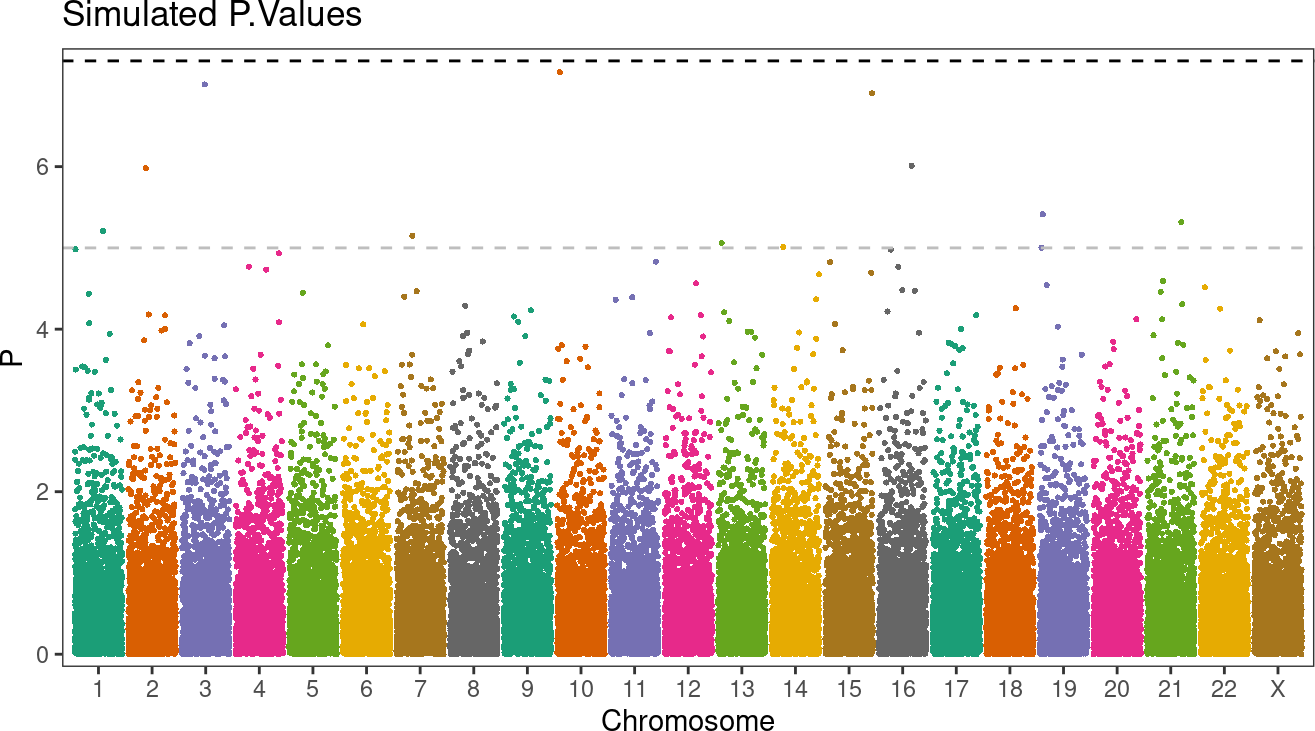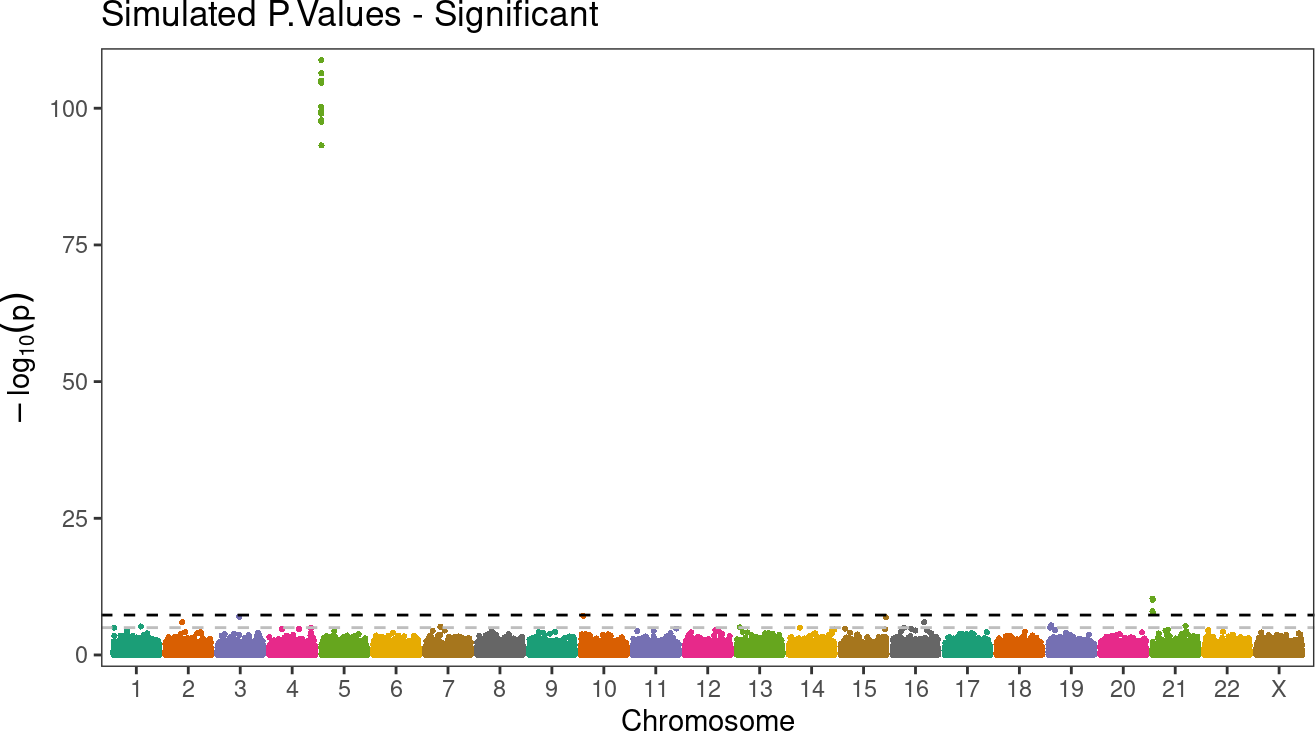# 1 Introduction

The package `ggmanh` is aimed to provide easy and direct access to visualisation to the GWAS / PWAS results while also providing many functionalities and features.

Manhattan plot is commonly used to display significant Single Nucleotide Polymorphisms (SNPs) in Genome Wide Association Study (GWAS). The x-axis is divided into chromosomes, and SNPs are plotted in their respective positions. The y-axis typically represents \(-10*log(p value)\). Majority of the points have low y-values, with some of the significant SNPs having high y-values. It is not uncommon to see strong association between SNPs and a phenotype, yielding a high y-value. This results in a wide y-scales in which SNPs with lower significance are squished.

This function addresses this problem by rescaling the y-axis according to the range of y. There are more features, such as labelling without overlap (with the help of ggprepel package), reflecting the size of chromosomes along the x-axis, and displaying significant lines.

The package can be installed by:

``````if (!require("BiocManager"))
install.packages("BiocManager")
BiocManager::install("ggmanh")``````

# 2 Functions Overview

`manhattan_plot()` is a generic method that can take a `data.frame`, `MPdata`, or a `GRanges` object. The `data.frame`, at bare minimum, must have three columns containing: `chromosome`, `position`, and `p.value`. For a `GRanges` object, meta data column name for the p-value needs to be passed.

There are two steps to this function: preprocess data and plot data. The preprocessing step (accomplished with `manhattan_data_preprocess()`) preprocesses the data by calculating the new x-position to map to the plot (`new_pos` column added to the data), “thining” the data points, and saving other graphical information needed for manhattan plot, which is returned as a `MPdata` object. The plot step (accomplished with `manhattan_plot()`) determines if rescaling of the y-axis is needed and plots / saves the manhattan plot.

While using `manhattan_plot()` on `data.frame` is sufficient, it is fine to separately run pre-processing and plotting for customizing the plot without having to preprocess again and again.

# 3 Example with Simulated GWAS

``````library(ggmanh)
library(SeqArray)

First, create a simulated data to be used for demonstration.

``````set.seed(1000)

nsim <- 50000

simdata <- data.frame(
"chromosome" = sample(c(1:22,"X"), size = nsim, replace = TRUE),
"position" = sample(1:100000000, size = nsim),
"P.value" = rbeta(nsim, shape1 = 5, shape2 = 1)^7
)``````

`manhattan_plot` expects data.frame to have at least three columns: chromosome, position, and p.value.

``````head(simdata)
#>   chromosome position     P.value
#> 1         16 41575779 0.135933290
#> 2          4 73447172 0.764033749
#> 3         11 82120979 0.002440878
#> 4         22 94419970 0.644460838
#> 5         19 38141341 0.184945910
#> 6          3 43235060 0.774330251``````

To avoid ambiguity in plotting, it is recommended that that the chromosome column is passed as a factor, or `chr.order` is specified.

``simdata\$chromosome <- factor(simdata\$chromosome, c(1:22,"X"))``

This is the bare minimum to plot manhattan plot, and `manhattan_plot` can handle the rest.

``````g <- manhattan_plot(x = simdata, pval.colname = "P.value", chr.colname = "chromosome", pos.colname = "position", plot.title = "Simulated P.Values", y.label = "P")
g```````manhattan_plot` is also defaulted to display the GWAS p.value threshold at `5e-8` and `5e-7`. For now, the threshold is required; the values and color can be customized.

## 3.1 Rescaling

The function is also suited to rescale the y-axis depending on the magnitude of p values.

Let’s suppose that there are signals from chromosome 5 and 21, and the significant p-value is low for chromosome 21 and even lower for chromosome 5.

``````tmpdata <- data.frame(
"chromosome" = c(rep(5, 10), rep(21, 5)),
"position" = c(sample(250000:250100, 10, replace = FALSE), sample(590000:600000, 5, replace = FALSE)),
"P.value" = c(10^-(rnorm(10, 100, 3)), 10^-rnorm(5, 9, 1))
)

simdata <- rbind(simdata, tmpdata)
simdata\$chromosome <- factor(simdata\$chromosome, c(1:22,"X"))``````
``````g <- manhattan_plot(x = simdata, pval.colname = "P.value", chr.colname = "chromosome", pos.colname = "position", plot.title = "Simulated P.Values - Significant", rescale = FALSE)
g``````The significant point at chromosome 5 has such a small p-value compared to other chromosomes that other significant poitns are less visible and the pattern at other chromosomes are masked. Rescaling attempts to fix this by changing the visual scale near the significant cutoff, removing the large white space in between.

``````g <- manhattan_plot(x = simdata, pval.colname = "P.value", chr.colname = "chromosome", pos.colname = "position", plot.title = "Simulated P.Values - Significant", rescale = TRUE)
g``````